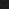JavaPerspective.com  >   Beginner Tutorials  >   3. Java Basics  >   3.8. Java arrays

3.8. Java arrays
Last updated: 23 January 2013.

An array is a variable which contains multiple values of the same type. An array has a fixed size that can't be changed after the array is created. The sample below declares an array of integers:

int[] myArray;

At this point, no memory is allocated to the array. To allocate memory to the array, you need to use the keyword new as shown below:

myArray = new int;

The above statement allocates enough memory for an array of 5 integers. The two statements above can be merged into a single statement:

int[] myArray = new int;

The initialization of the array elements can now be done this way:

myArray = 1;
myArray
 = 2;
myArray
 = 3;
myArray
 = 4;
myArray
 = 5;

As you can see, the index of the first element is 0 and the index of the last element is 4. In Java, array indexes start at 0.

You can also declare, allocate memory and initialize an array in a single statement like this:

int[] myArray = {1, 2, 3, 4, 5};

The code below prints all of the array elements to the standard output:

System.out.println(myArray);
System.out.println
(myArray);
System.out.println
(myArray);
System.out.println
(myArray);
System.out.println
(myArray);

The output is:

1
2
3
4
5

myArray.length gives you the size of the array. If you execute this line of code:

System.out.println(myArray.length);

You will get this output:

5

In the Java language, multidimensional arrays are declared as arrays of arrays:

int[][] myArray = new int;

myArray
 = new int[]{1, 2, 3, 4, 5};
myArray
 = new int[]{11, 22, 33, 44, 55};
myArray
 = new int[]{111, 222, 333, 444, 555};

System.out.println
(myArray);
System.out.println
(myArray);
System.out.println
(myArray);

The output is:

1
33
555

You are here :  JavaPerspective.com  >   Beginner Tutorials  >   3. Java Basics  >   3.8. Java arrays
Next tutorial :  JavaPerspective.com  >   Beginner Tutorials  >   3. Java Basics  >   3.9. The if-else statement